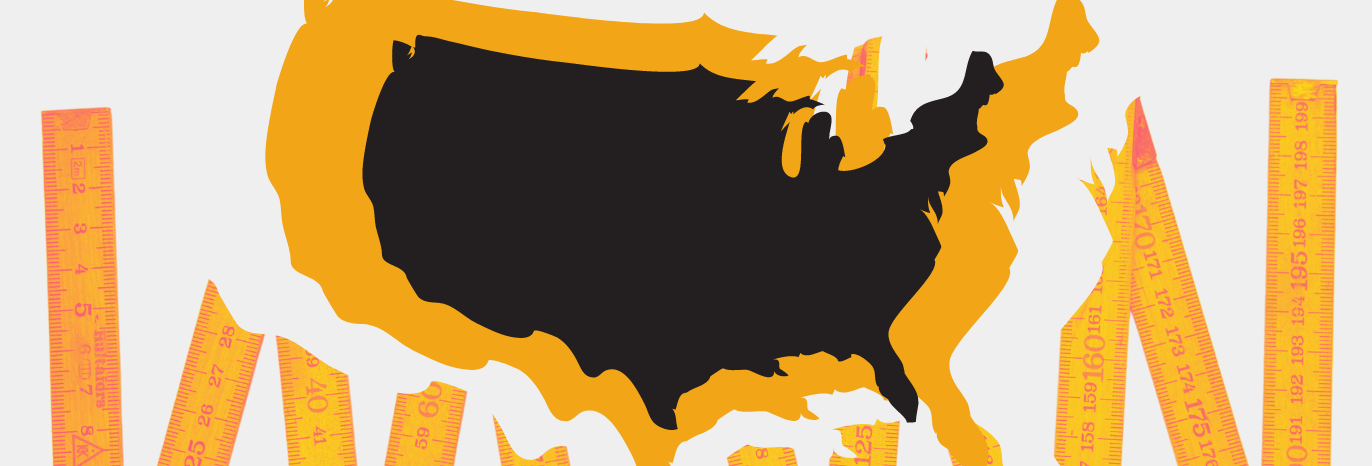# Going from the Metric System to the Imperial System in the United States

When I was a child, I sat at the 3rd desk in the 3rd row in Miss Sullivan’s 3rd-grade class. One morning, Miss Sullivan made an announcement. She said, “Listen, kids, everybody in the world is using the Metric System except for people here in the United States, so it’s about time we started learning it, too. By the time you all graduate from high school, the whole country will be on the Metric System.” Well, folks, that was a long, long time ago and, with the exception of 2-liter Coke bottles and a few other items, we haven’t even come close to going metric, and I don’t expect it to happen any time soon. Therefore, I strongly suggest that you become familiar with the Imperial System, which is what we use in the United States. Here are some guidelines.

In the United States, to measure things, we don’t use millimeters, centimeters, meters, or kilometers. We use inches, feet, yards, and miles, and how to say them is in parentheses on the right.

12"    =    12 in. (12 inches)

3'      =     3 ft.   (3 feet)

1 yd.  =    (1 yard)

12" = 1'  =  (12 inches equals one foot)

3' = 1 yd. =  (3 feet equals one yard)

6.2 mi. = 10 km (six point two miles equals ten kilometers)

When baking or cooking you must understand the following abbreviations because all American recipes use these measurements.

tsp. = teaspoon

tbs. = tablespoon

c.= cup

pt. = pint

qt.= quart

gal. = gallon

The easiest way to understand fluid ounces (fl. oz.) is to go to the supermarket and look at the milk and cream containers. They all come in these sizes.

1/2 pt. (half-pint) = 8 fl. oz.

1 pt. (one pint) = 16 fl. oz.

1 qt. (one quart) = 32 fl. oz.

1/2 gal. (half-gallon) = 64 fl. oz.

1 gal. (one gallon) = 128 fl. oz.

The following abbreviations have to do with weights. To give you a bit of perspective, one package of butter (4 sticks) weighs one pound.

oz. (ounce)

lb. (pound)

lbs. (pounds)

16 oz. = 1lb.

32 oz. = 2 lbs.

If you watch the news on TV in the United States, you’ll see that the temperatures are always given in Fahrenheit rather than Celsius.

• To convert temperatures in degrees from Fahrenheit to Celsius, subtract 32 and multiply by .5556.
• To convert temperatures in degrees from Celsius to Fahrenheit, multiply by 1.8 and add 32.

But who has time to do all that math? The temperatures below are what we live with about 90% of the time, so if you can remember these numbers, you'll be "close enough".

If the meteorologist says it's 60 degrees Fahrenheit, look at the chart below......it's about 15 or 16 degrees Celsius ---> "close enough".

C = Celsius

F = Fahrenheit

40°C = 104°F

30°C = 86°F

20°C = 68°F

10°C = 50°F

0°C = 32° F

## How many words do I need to know in order to speak English fluently?

Some of you out there may be wondering “How many words do I really need to know in order to speak...

## How to Practice Listening

How can I improve my listening skills? This question is one of the most asked questions I have...

## The Benefits of Reading Out Loud

Many people have told me that reading books is good. Well, I kind of agree with that statement....